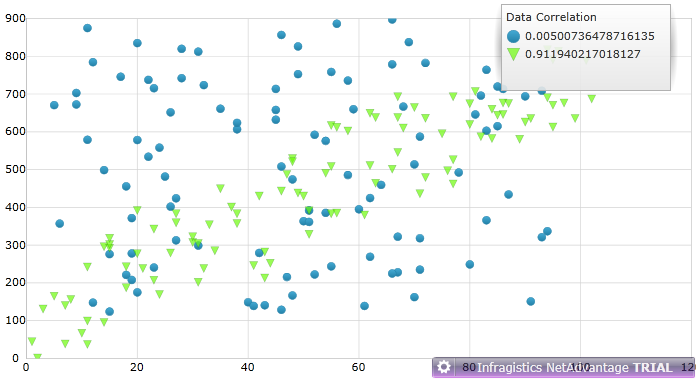# Introduction to Series Data Correlation with XamDataChart

Damyan Petev / Friday, November 11, 2011

This is one of the new features for you to take advantage of with the new release and it is a cooperation between the XamDataChart control and the CorrelationCalculator which is part of the Infragistics Math Calculators™ library. This integration provides a simple way to calculate correlation between two values in a data set displayed using the Scatter Series. The CorrelationCalculator calculates what is referred in statistics as the Pearson product-moment correlation coefficient - used to measure the linear association(correlation) between two variables (X and Y) in a data set with a value between +1 and −1. The closer to those marks the coefficient is the stronger the relationship between them. For example you can plot data for electricity usage spikes and cold weather and sure enough you will notice a dependence. If you are interested to read more on that you can find sufficient explanations in our documentation - especially the CorrelationCalculator page where you can find the formulas and see a comparison of types of correlation based on the coefficient with visual examples. And of course there’s an extensive article on Wikipedia right here.

## The Correlation calculator

Considering what it does this element doesn’t have a visual representation so naturally it would be defined in the sources section of the control, page or even the application. And since it calculates correlation it would require a data set(ItemsSource) and two variables to compare. Before we get to that as always you should add some assembly references – the shared InfragisticsSL4.v11.2.dll, then InfragisticsSL4.Math.v11.2.dll and InfragisticsSL4.Math.Calculators.v11.2.dll for the calculator and since we’ll use a XamDataChart you might as well add InfragisticsSL4.Controls.Charts.XamDataChart.v11.2.dll. Add the obligatory namespace in XAML:

1. xmlns:ig="http://schemas.infragistics.com/xaml"

At this point you set up the CorrelationCalculator and for presentation purposes we will use sample data generated by the CorrelationDataSample object, the code for which is made available in Visual Basic and C# on this page. Let’s add two different types of generated data and a calculator for each, bind its ItemsSource to them and provide paths for both values to be compared. In this case the generated data is created with X and Y:

1. <UserControl.Resources>
2.     <ResourceDictionary>
3.     <!--Random data-->
4.         <local:CorrelationDataSample x:Key="randomData">
5.             <local:CorrelationDataSample.Settings>
7.             </local:CorrelationDataSample.Settings>
8.         </local:CorrelationDataSample>
9.     <!--Data that is random and increasing-->
10.         <local:CorrelationDataSample x:Key="randomIncreasing">
11.             <local:CorrelationDataSample.Settings>
13.             </local:CorrelationDataSample.Settings>
14.         </local:CorrelationDataSample>
15.     <!--Define calculator for the random data-->
16.         <ig:CorrelationCalculator x:Key="correlCalc"
17.                               XMemberPath="X"
18.                               YMemberPath="Y"
19.                               ItemsSource="{StaticResource randomData}"/>
20.     <!--Define calculator for the randomly increasing data-->
21.         <ig:CorrelationCalculator x:Key="correlCalc2"
22.                               XMemberPath="X"
23.                               YMemberPath="Y"
24.                               ItemsSource="{StaticResource randomIncreasing}"/>
25.     </ResourceDictionary>
26. </UserControl.Resources>

## The Chart

At this point you have data, a calculator that has computed the correlation coefficient for the two members and all that is left is to display that data with simplicity and style using a XamDataChart Scatter series for each type of generated data. One efficient way to do that is bind the Title property of the series to the corresponding calculators’ Value:

1. <ig:XamDataChart Legend="{Binding ElementName=legend}">
2.     <ig:XamDataChart.Axes>
3.         <ig:NumericXAxis x:Name="xAxis" />
4.         <ig:NumericYAxis x:Name="yAxis" />
5.     </ig:XamDataChart.Axes>
6.     <ig:XamDataChart.Series>
7.         <ig:ScatterSeries
8.             Title="{Binding Value, Source={StaticResource correlCalc}}"
9.             ItemsSource="{StaticResource randomData}"
10.                           XMemberPath="X"
11.                           YMemberPath="Y"
12.                           XAxis="{Binding ElementName=xAxis}"
13.                           YAxis="{Binding ElementName=yAxis}"/>
14.         <ig:ScatterSeries
15.             Title="{Binding Value, Source={StaticResource correlCalc2}}"
16.             ItemsSource="{StaticResource randomIncreasing}"
17.                           XMemberPath="X"
18.                           YMemberPath="Y" XAxis="{Binding ElementName=xAxis}"
19.                           YAxis="{Binding ElementName=yAxis}" MarkerBrush="#DB8ED465" MarkerOutline="#54004B00" />
20.     </ig:XamDataChart.Series>
21. </ig:XamDataChart>

As you noticed on the very first row we defined a legend property for the chart so add a legend element too:

1. <ig:Legend Name="legend" Content="Data Correlation"/>

Then again the calculators’ results are available for you to display as you see fit but why would use something else when the end result can look like this:The blue series are the random data and the green are the randomly increasing data. As a side effect of using generated data you can actually tell how random ‘random’ really is - remember values closer to zero mean less correlation and this one is pretty low. I’ll admit this is one of the more close-to-perfect screenshots.

Check out our Silverlight online samples on this or download the offline WPF samples from here and if you want a project to try yourself – as always there’s one available here. The demo solution includes a Silverlight project and the same code in a WPF project just for the sake of reminding this is a XAML Cross-platform example after all.

Note: The solution has been cleaned so make sure the references are all in check and then build it.